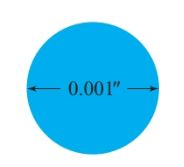Home / Basic Electrical / American Wire Gauge (AWG) System

# American Wire Gauge (AWG) System

Want create site? Find Free WordPress Themes and plugins.

Many conductors are much less than an inch in diameter. To simplify area calculations for such conductors, the foot-pound system uses an area unit, called a circular mil, based on a mil, or one-thousandth of an inch (see Figure 1).

Definition: One circular mil (CM) is the area of a circle 1 mil in diameter.Figure 1:  A circle with the diameter of one mil

When using circular mils, the formula for the area of a circle becomes

$\begin{matrix} A={{d}^{2}} & {} & \left( 1 \right) \\\end{matrix}$

Where A is the area of the circle in circular mils and d is the diameter in mils.

## Wire Size Example

Find the cross-sectional area of a wire 0.0280″ in diameter.

Solution

\begin{align} & d=0.02800”=28\text{ }mills \\ & A={{d}^{2}}={{28}^{2}}=784\text{ }CM \\\end{align}

## Resistivity Definition

Resistivity can be defined in terms of a piece of wire 1 ft long with a cross- sectional area of 1 CM, as shown in Figure 2. The volume of this wire is 1 circular-mil foot, and the units of ρ become CM-Ω/ft. Table 1 lists the resistivities for some metals.Figure 2 One circular-mil foot

 Material Resistivity Silver 9.56 Copper 10.4 Gold 14.0 Aluminum 17.0 Tungsten 34.0 Brass 42.0 Iron 61.0 Nichrome 675.0

Table 1 Specific Resistivities (${}^{\Omega }/{}_{c.mil-ft}$ at 20 oC)

## Resistance Calculation Example

Calculate the resistance of 50.0 miles of copper wire 0.3500 in diameter.

Solution

\begin{align} & d=0.350”=35mils \\ & A={{d}^{2}}=122.5CM \\ & l=50miles\times \frac{5280ft}{1mile}=2.54\times {{10}^{5}}ft \\ & R=\rho \frac{l}{A}=\frac{10.36\times \left( 2.54\times {{10}^{5}} \right)}{122.5\times {{10}^{3}}}=22.4\Omega \\\end{align}

## American Wire Gauge System

Definition: The system of notation for measuring the size of conductors or wires.

In North America, the most wire is produced in the standard American Wire Gauge (AWG) sizes listed in Table 2. The larger the gauge number, the smaller the wire size.

Wires larger than 4/0 (sometimes called 0000) are usually specified in thousands of circular mils (MCM) instead of having a gauge number.

The ratio of the diameter of adjacent American Wire Gauge sizes is about 1.1229, and the ratio of their areas is about 1.26.

The area ratio between wire sizes three AWG numbers apart is very close to 2. For example, #10 wire (10 380 CM) has about twice the area of #13 wire (5178 CM) and half the area of #7 wire (20 820 CM).

Since resistance is inversely proportional to area, the resistance per unit length also differs by a factor of about two for wires three AWG numbers apart. For example, #1 wire (0.1239 Ω/1000’) has half the resistance of #4 wire (0.2485 Ω/1000’) and twice the resistance of #3/0 wire (0.0618 Ω/1000’).

## Wire Size Calculation Example

What size of aluminum wire has a resistance of 1.62 Ω for a length of 2500’?

Solution

Since,

$R=\rho \frac{l}{A}$

So,

$A=\rho \frac{l}{R}=17\times \frac{2500}{1.62}=26.2MCM$

From the Table 2, we see that this area matches AWG #6.

 Gauge Number Diameter (Mils) Circular Mil Area Ohms Per 1000 Ft 00 365 133,000 0.0795 0 325 106,000 0.100 1 289 83,700 0.126 2 258 66,400 0.159 3 229 52,600 0.201 4 204 41,700 0.253 5 182 33,100 0.319 6 162 26,300 0.403 7 144 20,800 0.508 8 128 16,500 0.641 9 114 13,100 0.808 10 102 10,400 1.02 11 91 8,230 1.28 12 81 6,530 1.62 13 72 5,180 2.04 14 64 4,110 2.58 15 57 3,260 3.25 16 51 2,580 4.09 17 45 2,050 5.16 18 40 1,620 6.51 19 36 1,290 8.21 20 32 1,020 10.4 21 28.5 810 13.1 22 25.3 642 16.5 23 22.6 509 20.8 24 20.1 404 26.2 25 17.9 320 33 26 15.9 254 41.6 27 14.2 202 52.5 28 12.6 160 66.2 29 11.3 127 83.4 30 10.0 101 105 31 8.9 79.7 133 32 8.0 63.2 167 33 7.1 50.1 211 34 6.3 39.8 266 35 5.6 31.5 335 36 5.0 25.0 423

Table 2 American Wire Gauge (AWG) Wire Sizes Chart

Did you find apk for android? You can find new Free Android Games and apps.